pandas_pandas excel - CSDN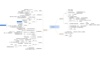pandas 订阅
pandas 是基于NumPy 的一种工具，该工具是为了解决数据分析任务而创建的。Pandas 纳入了大量库和一些标准的数据模型，提供了高效地操作大型数据集所需的工具。pandas提供了大量能使我们快速便捷地处理数据的函数和方法。你很快就会发现，它是使Python成为强大而高效的数据分析环境的重要因素之一。 展开全文pandas 是基于NumPy 的一种工具，该工具是为了解决数据分析任务而创建的。Pandas 纳入了大量库和一些标准的数据模型，提供了高效地操作大型数据集所需的工具。pandas提供了大量能使我们快速便捷地处理数据的函数和方法。你很快就会发现，它是使Python成为强大而高效的数据分析环境的重要因素之一。

pandas

PyData开发组

Python数据分析模块

AQR Capital Management
pandas简介
Pandas   是python的一个数据分析包，最初由AQR Capital Management于2008年4月开发，并于2009年底开源出来，目前由专注于Python数据包开发的PyData开发team继续开发和维护，属于PyData项目的一部分。Pandas最初被作为金融数据分析工具而开发出来，因此，pandas为时间序列分析提供了很好的支持。 Pandas的名称来自于面板数据（panel data）和python数据分析（data analysis）。panel data是经济学中关于多维数据集的一个术语，在Pandas中也提供了panel的数据类型。

• ## pandas用法大全

万次阅读 多人点赞 2017-09-08 15:51:38
1、首先导入pandas库，一般都会用到numpy库，所以我们先导入备用： import numpy as np import pandas as pd 2、导入CSV或者xlsx文件： df = pd.DataFrame(pd.read_csv(‘name.csv’,header=1)) df = pd....
一、生成数据表
1、首先导入pandas库，一般都会用到numpy库，所以我们先导入备用：

import numpy as np
import pandas as pd

2、导入CSV或者xlsx文件：

df = pd.DataFrame(pd.read_csv('name.csv',header=1))


3、用pandas创建数据表：

df = pd.DataFrame({"id":[1001,1002,1003,1004,1005,1006],
"date":pd.date_range('20130102', periods=6),
"city":['Beijing ', 'SH', ' guangzhou ', 'Shenzhen', 'shanghai', 'BEIJING '],
"age":[23,44,54,32,34,32],
"category":['100-A','100-B','110-A','110-C','210-A','130-F'],
"price":[1200,np.nan,2133,5433,np.nan,4432]},
columns =['id','date','city','category','age','price'])


2、数据表信息查看
1、维度查看：

df.shape


2、数据表基本信息（维度、列名称、数据格式、所占空间等）：

df.info()


3、每一列数据的格式：

df.dtypes


4、某一列格式：

df['B'].dtype


5、空值：

df.isnull()


6、查看某一列空值：

df.isnull()


7、查看某一列的唯一值：

df['B'].unique()


8、查看数据表的值：
df.values
9、查看列名称：

df.columns


10、查看前10行数据、后10行数据：

df.head() #默认前10行数据
df.tail()    #默认后10 行数据


三、数据表清洗
1、用数字0填充空值：

df.fillna(value=0)


2、使用列prince的均值对NA进行填充：

df['prince'].fillna(df['prince'].mean())


3、清楚city字段的字符空格：

df['city']=df['city'].map(str.strip)


4、大小写转换：

df['city']=df['city'].str.lower()


5、更改数据格式：

df['price'].astype('int')


6、更改列名称：

df.rename(columns={'category': 'category-size'})


7、删除后出现的重复值：

df['city'].drop_duplicates()


8、删除先出现的重复值：

df['city'].drop_duplicates(keep='last')


9、数据替换：

df['city'].replace('sh', 'shanghai')


四、数据预处理

df1=pd.DataFrame({"id":[1001,1002,1003,1004,1005,1006,1007,1008],
"gender":['male','female','male','female','male','female','male','female'],
"pay":['Y','N','Y','Y','N','Y','N','Y',],
"m-point":[10,12,20,40,40,40,30,20]})


1、数据表合并

df_inner=pd.merge(df,df1,how='inner')  # 匹配合并，交集
df_left=pd.merge(df,df1,how='left')        #
df_right=pd.merge(df,df1,how='right')
df_outer=pd.merge(df,df1,how='outer')  #并集


2、设置索引列

df_inner.set_index('id')


3、按照特定列的值排序：

df_inner.sort_values(by=['age'])


4、按照索引列排序：

df_inner.sort_index()


5、如果prince列的值>3000，group列显示high，否则显示low：

df_inner['group'] = np.where(df_inner['price'] > 3000,'high','low')


6、对复合多个条件的数据进行分组标记

df_inner.loc[(df_inner['city'] == 'beijing') & (df_inner['price'] >= 4000), 'sign']=1


7、对category字段的值依次进行分列，并创建数据表，索引值为df_inner的索引列，列名称为category和size

pd.DataFrame((x.split('-') for x in df_inner['category']),index=df_inner.index,columns=['category','size']))


8、将完成分裂后的数据表和原df_inner数据表进行匹配

df_inner=pd.merge(df_inner,split,right_index=True, left_index=True)


五、数据提取
主要用到的三个函数：loc,iloc和ix，loc函数按标签值进行提取，iloc按位置进行提取，ix可以同时按标签和位置进行提取。
1、按索引提取单行的数值

df_inner.loc


2、按索引提取区域行数值

df_inner.iloc[0:5]


3、重设索引

df_inner.reset_index()


4、设置日期为索引

df_inner=df_inner.set_index('date')


5、提取4日之前的所有数据

df_inner[:'2013-01-04']


6、使用iloc按位置区域提取数据

df_inner.iloc[:3,:2] #冒号前后的数字不再是索引的标签名称，而是数据所在的位置，从0开始，前三行，前两列。


7、适应iloc按位置单独提起数据

df_inner.iloc[[0,2,5],[4,5]] #提取第0、2、5行，4、5列


8、使用ix按索引标签和位置混合提取数据

df_inner.ix[:'2013-01-03',:4] #2013-01-03号之前，前四列数据


9、判断city列的值是否为北京

df_inner['city'].isin(['beijing'])


10、判断city列里是否包含beijing和shanghai，然后将符合条件的数据提取出来

df_inner.loc[df_inner['city'].isin(['beijing','shanghai'])]


11、提取前三个字符，并生成数据表

pd.DataFrame(category.str[:3])


六、数据筛选
使用与、或、非三个条件配合大于、小于、等于对数据进行筛选，并进行计数和求和。
1、使用“与”进行筛选

df_inner.loc[(df_inner['age'] > 25) & (df_inner['city'] == 'beijing'), ['id','city','age','category','gender']]


2、使用“或”进行筛选

df_inner.loc[(df_inner['age'] > 25) | (df_inner['city'] == 'beijing'), ['id','city','age','category','gender']].sort(['age'])


3、使用“非”条件进行筛选

df_inner.loc[(df_inner['city'] != 'beijing'), ['id','city','age','category','gender']].sort(['id'])


4、对筛选后的数据按city列进行计数

df_inner.loc[(df_inner['city'] != 'beijing'), ['id','city','age','category','gender']].sort(['id']).city.count()


5、使用query函数进行筛选

df_inner.query('city == ["beijing", "shanghai"]')


6、对筛选后的结果按prince进行求和

df_inner.query('city == ["beijing", "shanghai"]').price.sum()


七、数据汇总
主要函数是groupby和pivote_table
1、对所有的列进行计数汇总

df_inner.groupby('city').count()


2、按城市对id字段进行计数

df_inner.groupby('city')['id'].count()


3、对两个字段进行汇总计数

df_inner.groupby(['city','size'])['id'].count()


4、对city字段进行汇总，并分别计算prince的合计和均值

df_inner.groupby('city')['price'].agg([len,np.sum, np.mean])


八、数据统计
数据采样，计算标准差，协方差和相关系数
1、简单的数据采样

df_inner.sample(n=3)


2、手动设置采样权重

weights = [0, 0, 0, 0, 0.5, 0.5]
df_inner.sample(n=2, weights=weights)


3、采样后不放回

df_inner.sample(n=6, replace=False)


4、采样后放回

df_inner.sample(n=6, replace=True)


5、 数据表描述性统计

df_inner.describe().round(2).T #round函数设置显示小数位，T表示转置


6、计算列的标准差

df_inner['price'].std()


7、计算两个字段间的协方差

df_inner['price'].cov(df_inner['m-point'])


8、数据表中所有字段间的协方差

df_inner.cov()


9、两个字段的相关性分析

df_inner['price'].corr(df_inner['m-point']) #相关系数在-1到1之间，接近1为正相关，接近-1为负相关，0为不相关


10、数据表的相关性分析

df_inner.corr()


九、数据输出
分析后的数据可以输出为xlsx格式和csv格式
1、写入Excel

df_inner.to_excel('excel_to_python.xlsx', sheet_name='bluewhale_cc')


2、写入到CSV

df_inner.to_csv('excel_to_python.csv')

展开全文pandas
• Pandas包是基于Python平台的数据管理利器，已经成为了Python进行数据分析和挖掘时的数据基础平台和事实上的工业标准，掌握其使用方法，是使用Python进行数据分析和数据挖掘的必备条件。  本课程通过讲解Pandas基础...python工程师 python爬虫 python python教程 python安装
• ## pandas用法总结

万次阅读 多人点赞 2020-06-11 16:11:25
1、首先导入pandas库，一般都会用到numpy库，所以我们先导入备用： import numpy as np import pandas as pd 2、导入CSV或者xlsx文件： df = pd.DataFrame(pd.read_csv(‘name.csv’,header=1)) df = pd....
一、生成数据表
1、首先导入pandas库，一般都会用到numpy库，所以我们先导入备用：
import numpy as np
import pandas as pd

2、导入CSV或者xlsx文件：
df = pd.DataFrame(pd.read_csv('name.csv',header=1))

或者
import pandas as pd
from collections import namedtuple

items = []

with codecs.open('reply.pv.07', 'r', 'utf-8') as f:
for line in f:
line_split = line.strip().split('\t')
items.append(Item(line_split.strip(), line_split.strip()))


3、用pandas创建数据表：
df = pd.DataFrame({"id":[1001,1002,1003,1004,1005,1006],
"date":pd.date_range('20130102', periods=6),
"city":['Beijing ', 'SH', ' guangzhou ', 'Shenzhen', 'shanghai', 'BEIJING '],
"age":[23,44,54,32,34,32],
"category":['100-A','100-B','110-A','110-C','210-A','130-F'],
"price":[1200,np.nan,2133,5433,np.nan,4432]},
columns =['id','date','city','category','age','price'])

二、数据表信息查看
1、维度查看：
df.shape

2、数据表基本信息（维度、列名称、数据格式、所占空间等）：
df.info()

3、每一列数据的格式：
df.dtypes

4、某一列格式：
df['B'].dtype

5、空值：
df.isnull()

6、查看某一列空值：
df.isnull()

7、查看某一列的唯一值：
df['B'].unique()

8、查看数据表的值：
df.values

9、查看列名称：
df.columns

10、查看前5行数据、后5行数据：
df.head() #默认前5行数据
df.tail()    #默认后5行数据

三、数据表清洗
1、用数字0填充空值：
df.fillna(value=0)

2、使用列prince的均值对NA进行填充：
df['prince'].fillna(df['prince'].mean())

3、清楚city字段的字符空格：
df['city']=df['city'].map(str.strip)

4、大小写转换：
df['city']=df['city'].str.lower()

5、更改数据格式：
df['price'].astype('int')

6、更改列名称：
df.rename(columns={'category': 'category-size'})

7、删除后出现的重复值：
df['city'].drop_duplicates()

8 、删除先出现的重复值：
df['city'].drop_duplicates(keep='last')

9、数据替换：
df['city'].replace('sh', 'shanghai')

四、数据预处理
df1=pd.DataFrame({"id":[1001,1002,1003,1004,1005,1006,1007,1008],
"gender":['male','female','male','female','male','female','male','female'],
"pay":['Y','N','Y','Y','N','Y','N','Y',],
"m-point":[10,12,20,40,40,40,30,20]})

1、数据表合并
1.1 merge
df_inner=pd.merge(df,df1,how='inner')  # 匹配合并，交集
df_left=pd.merge(df,df1,how='left')        #
df_right=pd.merge(df,df1,how='right')
df_outer=pd.merge(df,df1,how='outer')  #并集

1.2 append
result = df1.append(df2)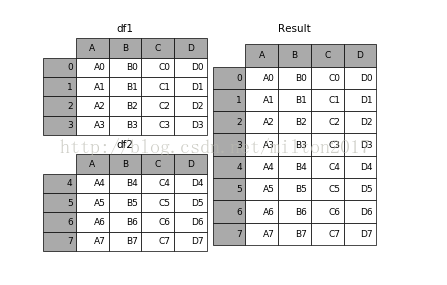1.3 join
result = left.join(right, on='key')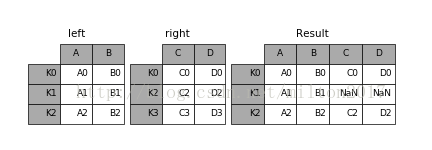1.4 concat
pd.concat(objs, axis=0, join='outer', join_axes=None, ignore_index=False,
keys=None, levels=None, names=None, verify_integrity=False,
copy=True)


objs︰ 一个序列或系列、 综合或面板对象的映射。如果字典中传递，将作为键参数，使用排序的键，除非它传递，在这种情况下的值将会选择
（见下文）。任何没有任何反对将默默地被丢弃，除非他们都没有在这种情况下将引发 ValueError。
axis: {0，1，…}，默认值为 0。要连接沿轴。
join: {‘内部’、 ‘外’}，默认 ‘外’。如何处理其他 axis(es) 上的索引。联盟内、 外的交叉口。
ignore_index︰ 布尔值、 默认 False。如果为 True，则不要串联轴上使用的索引值。由此产生的轴将标记
0，…，n-1。这是有用的如果你串联串联轴没有有意义的索引信息的对象。请注意在联接中仍然受到尊重的其他轴上的索引值。
join_axes︰ 索引对象的列表。具体的指标，用于其他 n-1 轴而不是执行内部/外部设置逻辑。 keys︰
序列，默认为无。构建分层索引使用通过的键作为最外面的级别。如果多个级别获得通过，应包含元组。
levels︰ 列表的序列，默认为无。具体水平 （唯一值） 用于构建多重。否则，他们将推断钥匙。
names︰ 列表中，默认为无。由此产生的分层索引中的级的名称。
verify_integrity︰ 布尔值、 默认 False。检查是否新的串联的轴包含重复项。这可以是相对于实际数据串联非常昂贵。
副本︰ 布尔值、 默认 True。如果为 False，请不要，不必要地复制数据。

例子：1.frames = [df1, df2, df3]
2.result = pd.concat(frames)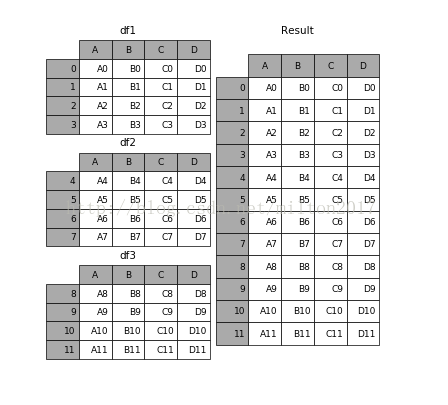2、设置索引列
df_inner.set_index('id')

3、按照特定列的值排序：
df_inner.sort_values(by=['age'])

4、按照索引列排序：
df_inner.sort_index()

5、如果prince列的值>3000，group列显示high，否则显示low：
df_inner['group'] = np.where(df_inner['price'] > 3000,'high','low')

6、对复合多个条件的数据进行分组标记
df_inner.loc[(df_inner['city'] == 'beijing') & (df_inner['price'] >= 4000), 'sign']=1

7、对category字段的值依次进行分列，并创建数据表，索引值为df_inner的索引列，列名称为category和size
pd.DataFrame((x.split('-') for x in df_inner['category']),index=df_inner.index,columns=['category','size']))

8、将完成分裂后的数据表和原df_inner数据表进行匹配
df_inner=pd.merge(df_inner,split,right_index=True, left_index=True)

五、数据提取
主要用到的三个函数：loc,iloc和ix，loc函数按标签值进行提取，iloc按位置进行提取，ix可以同时按标签和位置进行提取。
1、按索引提取单行的数值
df_inner.loc

2、按索引提取区域行数值
df_inner.iloc[0:5]

3、重设索引
df_inner.reset_index()

4、设置日期为索引
df_inner=df_inner.set_index('date')

5、提取4日之前的所有数据
df_inner[:'2013-01-04']

6、使用iloc按位置区域提取数据
df_inner.iloc[:3,:2] #冒号前后的数字不再是索引的标签名称，而是数据所在的位置，从0开始，前三行，前两列。

7、适应iloc按位置单独提起数据
df_inner.iloc[[0,2,5],[4,5]] #提取第0、2、5行，4、5列

8、使用ix按索引标签和位置混合提取数据
df_inner.ix[:'2013-01-03',:4] #2013-01-03号之前，前四列数据

9、判断city列的值是否为北京
df_inner['city'].isin(['beijing'])

10、判断city列里是否包含beijing和shanghai，然后将符合条件的数据提取出来
df_inner.loc[df_inner['city'].isin(['beijing','shanghai'])]

11、提取前三个字符，并生成数据表
pd.DataFrame(category.str[:3])

六、数据筛选
使用与、或、非三个条件配合大于、小于、等于对数据进行筛选，并进行计数和求和。
1、使用“与”进行筛选
df_inner.loc[(df_inner['age'] > 25) & (df_inner['city'] == 'beijing'), ['id','city','age','category','gender']]

2、使用“或”进行筛选
df_inner.loc[(df_inner['age'] > 25) | (df_inner['city'] == 'beijing'), ['id','city','age','category','gender']].sort(['age'])

3、使用“非”条件进行筛选
df_inner.loc[(df_inner['city'] != 'beijing'), ['id','city','age','category','gender']].sort(['id'])

4、对筛选后的数据按city列进行计数
df_inner.loc[(df_inner['city'] != 'beijing'), ['id','city','age','category','gender']].sort(['id']).city.count()

5、使用query函数进行筛选
df_inner.query('city == ["beijing", "shanghai"]')

6、对筛选后的结果按prince进行求和
df_inner.query('city == ["beijing", "shanghai"]').price.sum()

七、数据汇总
主要函数是groupby和pivote_table
1、对所有的列进行计数汇总
df_inner.groupby('city').count()

2、按城市对id字段进行计数
df_inner.groupby('city')['id'].count()

3、对两个字段进行汇总计数
df_inner.groupby(['city','size'])['id'].count()

4、对city字段进行汇总，并分别计算prince的合计和均值
df_inner.groupby('city')['price'].agg([len,np.sum, np.mean])

八、数据统计
数据采样，计算标准差，协方差和相关系数
1、简单的数据采样
df_inner.sample(n=3)

2、手动设置采样权重
weights = [0, 0, 0, 0, 0.5, 0.5]
df_inner.sample(n=2, weights=weights)

3、采样后不放回
df_inner.sample(n=6, replace=False)

4、采样后放回
df_inner.sample(n=6, replace=True)

5、 数据表描述性统计
df_inner.describe().round(2).T #round函数设置显示小数位，T表示转置

6、计算列的标准差
df_inner['price'].std()

7、计算两个字段间的协方差
df_inner['price'].cov(df_inner['m-point'])

8、数据表中所有字段间的协方差
df_inner.cov()

9、两个字段的相关性分析
df_inner['price'].corr(df_inner['m-point']) #相关系数在-1到1之间，接近1为正相关，接近-1为负相关，0为不相关

10、数据表的相关性分析
df_inner.corr()

九、数据输出
分析后的数据可以输出为xlsx格式和csv格式
1、写入Excel
df_inner.to_excel('excel_to_python.xlsx', sheet_name='bluewhale_cc')

2、写入到CSV
df_inner.to_csv('excel_to_python.csv')



展开全文pandas python
• ## 【数据处理与分析】pandas快速入门

千次阅读 多人点赞 2019-06-03 12:43:18
参考教程：点这里 目录 pandas的series和dataFrame pandas选择数据 pandas设置值 ...pandas处理丢失数据NaN ...pandas导入导出 ...pandas数据合并 ...pandas和numpy的关系：numpy是列表，pandas是字典，pandas基于...

参考教程：点这里

目录

pandas的series和dataFrame

pandas选择数据

pandas设置值

pandas处理丢失数据NaN

pandas导入导出

pandas数据合并

pandas结合plot绘图

END

pandas的series和dataFrame

pandas和numpy的关系：numpy是列表，pandas是字典，pandas基于numpy构建。

Series的形式：索引在左边，值在右边。没有为数据指定索引会自动创建0到N-1（N为长度）的整数型索引。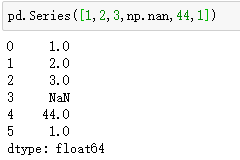DataFrame是一个表格型的数据结构，每列可以是不同的值类型，既有行索引也有列索引。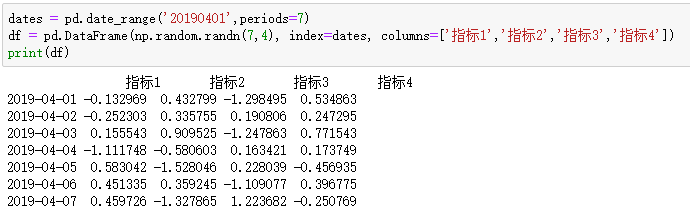取df的“指标1”列：df['指标1']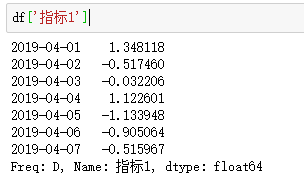创建一组没有给定行标签和列标签的数据：pd.DataFrame(np.arange(12).reshape((3,4)))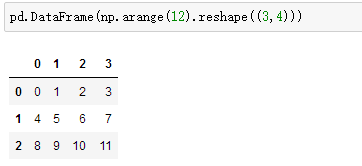创建对每一列的数据进行特殊对待的数据：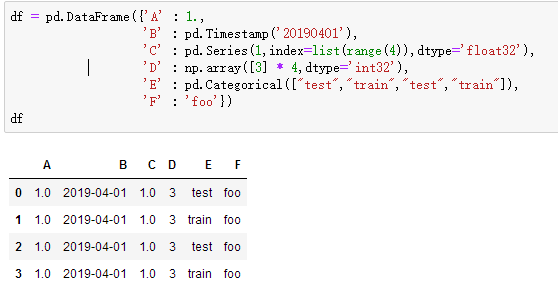查看数据类型：df.dtypes

查看索引（行）的序号：df.index

查看每种数据（列）的名：df.columns

查看df的所有值：df.values

查看数据的总结（计数、平均值、最值等）：df.describe()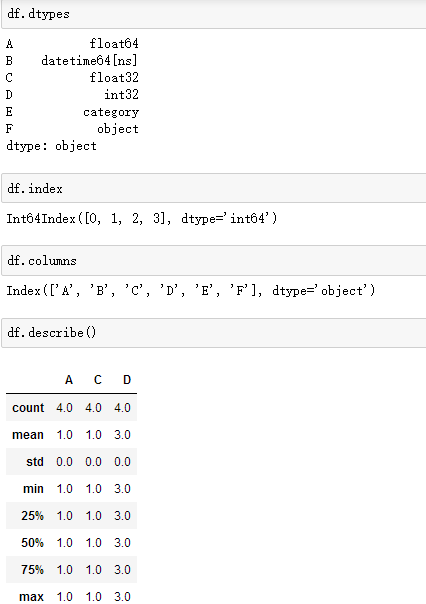翻转数据：df.T

对数据的 index 进行排序：df.sort_index(axis=1, ascending=False)

对数据的 值 进行排序：df.sort_values(by='A')

pandas选择数据

选择某列A： df['A'] 或 df.A

选择0到2多列：df[0:2]

根据标签选择1行：df.loc 【选择索引为0的一行】

选择所有行（: 代表所有行）：df.loc[:,['A','B']]  【选择所有行的A、B两列】

df.loc[3,['A','B']] 【选择第3行的A、B两列】

根据位置（索引）进行选择数据：df.iloc[3,1]  【第3行第1列的数据】

df.iloc[2:3,0:3]   【第2到3行，第0到3列的数据】

df.iloc[[0,3],0:3] 【第0、3行，第0到3列的数据】

通过判断的筛选：df[df.A==2]  【选择列A的值为2的行】

pandas设置值

利用索引：df.iloc[2,2] = 1111  【修改第2行、 第2列】

利用标签：df.loc['20190401','B'] = 2222  【修改行‘20190401’、 列‘B’】

根据条件：df.B[df.A>4] = 0  【列A大于4的都改成0】

按行或列：df['F'] = np.nan  【加上新列F，并设值为NaN】

df_original['title_keywords'] = ['' for _ in range(content_num)]

添加数据：df['G'] = pd.Series([1,2,3,4,5,6], index=pd.date_range('20190401',periods=6))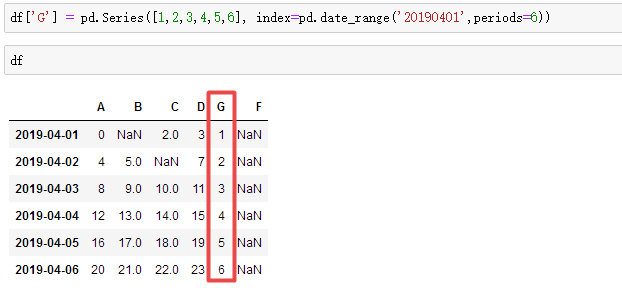pandas处理丢失数据NaN

直接去掉有 NaN 的行或列（pd.dropna()）：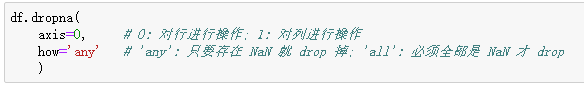将 NaN 的值用其他值代替（pd.fillna()）：df.fillna(value=0)  【全替换成0】

判断每个值是否是缺失数据：df.isnull()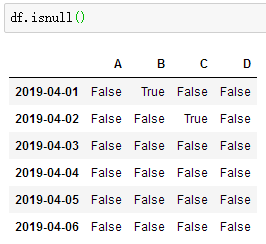检测整个数据表中是否存在 NaN, 如果存在就返回 True：np.any(df.isnull()) == True

pandas导入导出

pandas可以读取与存取的格式：csv、excel、json、html、pickle等 【官方文档】

将资料存取成pickle：data.to_pickle('student.pickle')

pandas数据合并

concat纵向合并：res = pd.concat([df1, df2, df3], axis=0)  【可以发现索引没变】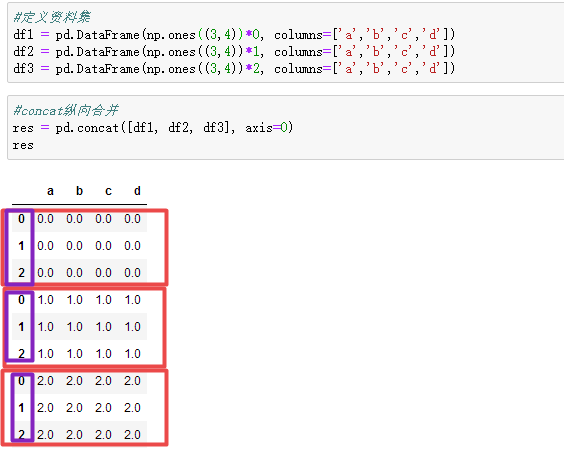concat参数之ignore_index (重置 index) ：res = pd.concat([df1, df2, df3], axis=0, ignore_index=True)

concat参数之join (合并方式，默认join='outer') ：res = pd.concat([df1, df2], axis=0, join='outer')  【依照column来做纵向合                                                                                                      并，有相同的column上下合并在一起，其他独自的column个自成列，原本                                                                                                  没有值的位置皆以NaN填充。】

concat参数之join (合并方式，改为join='inner') ：res = pd.concat([df1, df2], axis=0, join='inner')   【只有相同的column合并                                                                                                    在一起，其他的会被抛弃】

concat参数之join_axes：【根据df1的行索引来join，下图2为去掉join_axes】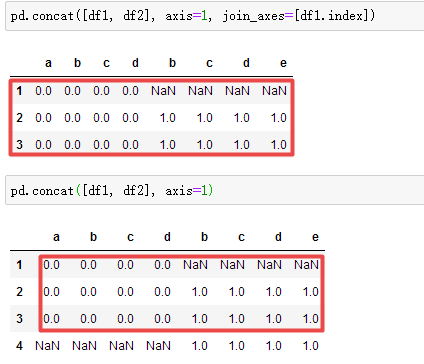append只有纵向合并，没有横向合并：

df1.append(df2, ignore_index=True)  【将df2合并到df1的下面，重置index】

df1.append([df2, df3], ignore_index=True)  【合并多个df，将df2与df3合并至df1的下面，重置index】

df1.append(s1, ignore_index=True)  【合并series，将s1合并至df1，重置index】

merge用于两组有key column的数据【样例数据如下】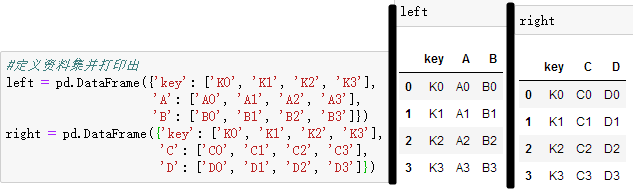依据key1与key2 columns进行合并，并打印出四种结果['left', 'right', 'outer', 'inner']：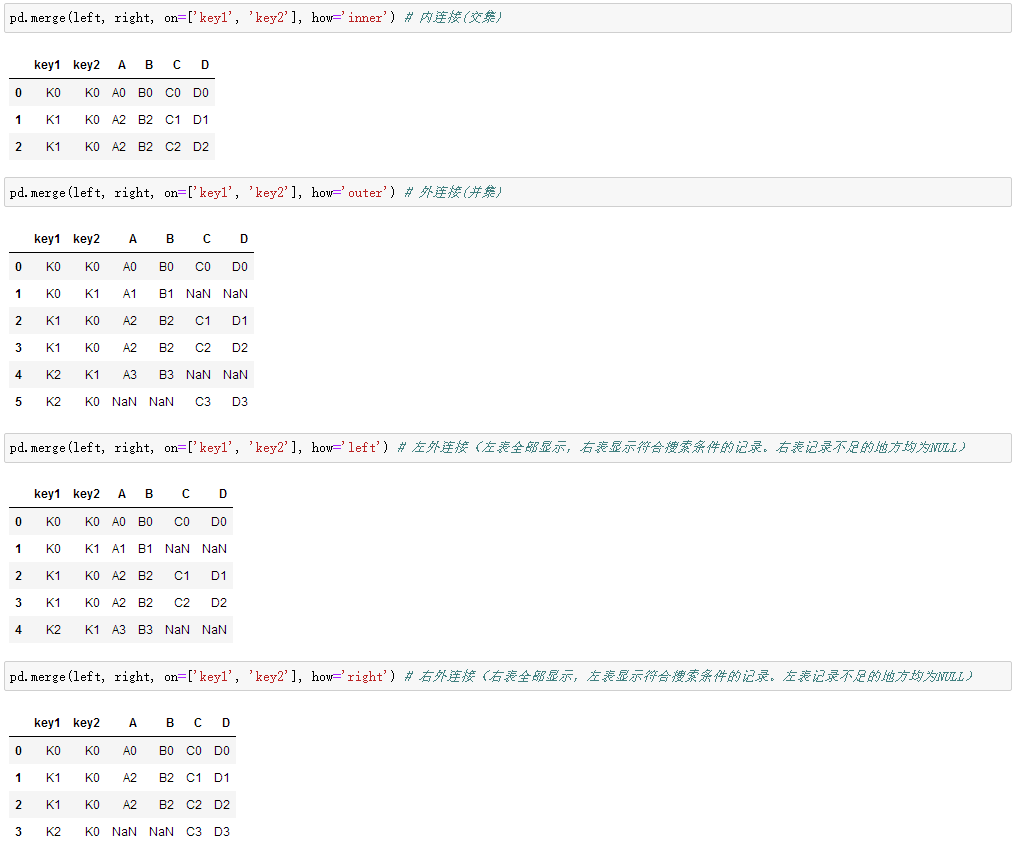merge参数之indicator=True会将合并的记录放在新的一列

merge参数之indicator=str，自定义列名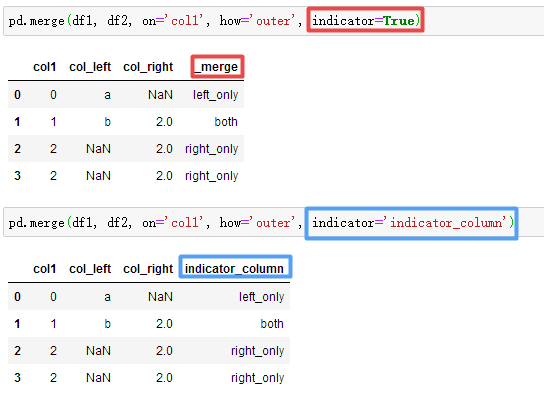根据index合并：res = pd.merge(left, right, left_index=True, right_index=True, how='outer')

merge参数之suffixes解决overlapping（重叠）的问题 【名为K0的有一个1岁男、1个4岁女、1个5岁女】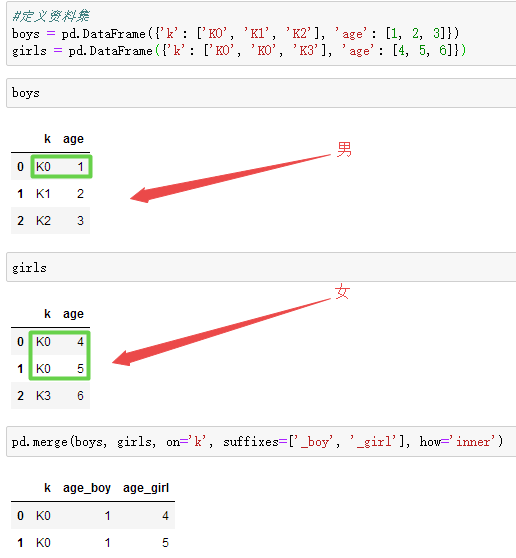pandas结合plot绘图

import matplotlib.pyplot as plt


随机生成1000个数据，Series 默认的 index 就是从0开始的整数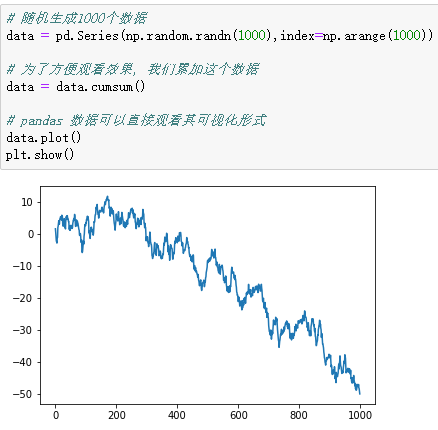生成一个1000*4 的DataFrame，并对他们累加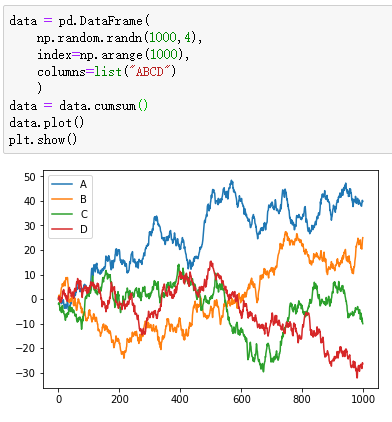散点图scatter只有x，y两个属性，我们我们就可以分别给x, y指定数据【下图line1】

再画一个在同一个ax上面，选择不一样的数据列，不同的 color 和 label【下图line2】

显示图片【下图line3】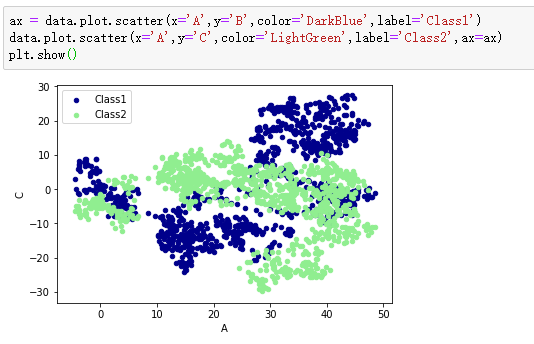END


展开全文pandas
• ## 什么是 Pandas?

千次阅读 2018-11-10 16:52:05
什么是 Pandas? Pandas 是 Python 的外部模块，它非常像 Excel，提供了分析数据的功能。它提供了两个数据类型 Series 和 DataFrame。 什么是 Series？ Series 是 Pandas 提供的一种数据类型，你可以把它想象成 Excel...
– Start
什么是 Pandas?
Pandas 是 Python 的外部模块，它非常像 Excel，提供了分析数据的功能。它提供了两个数据类型 Series 和 DataFrame。
什么是 Series？
Series 是 Pandas 提供的一种数据类型，你可以把它想象成 Excel 的一行或一列。
import numpy as np
import pandas as pd

# 创建 Series，pandas 自动创建 index
s = pd.Series([1, 3, 5, np.nan, 6, 8])
print(s)


什么是 DataFrame？
DataFrame 是 Pandas 提供的一种数据类型，你可以把它想象成 Excel 的表格。
import numpy as np
import pandas as pd

# 创建数据集
data = np.random.randn(6, 4)

# 创建 DataFrame
df = pd.DataFrame(data)
print(df)

– 更多参见：Pandas 精萃
– 声 明：转载请注明出处
– Last Updated on 2018-11-10
– Written by ShangBo on 2018-10-29
– End


展开全文• Python之所以适合数据分析，是因为它有很多第三方强大的库来协助，pandas就是其中之一。pandas的文档中是这样描述的： “快速，灵活，富有表现力的数据结构，旨在使”关系“或”标记“数据的使用既简单又直观。” ...Pandas
• 作者：luanhz来源：小数志导读本文主要是对pandas进行入门详细介绍，通过本文你将系统性了解pandas为何会有数据分析界"瑞士军刀"的盛誉。行文二级目录01 关于...
• ## 十分钟搞定pandas

万次阅读 多人点赞 2018-06-23 16:04:17
【原】十分钟搞定pandas本文是对pandas官方网站上《10 Minutes to pandas》的一个简单的翻译，原文在这里。这篇文章是对pandas的一个简单的介绍，详细的介绍请参考：Cookbook 。习惯上，我们会按下面格式引入所需要...
• pandas的数据 结构：Series、DataFrame、索引对象 pandas基本功能：重新索引，丢弃指定轴上的项，索引、选取和过滤，算术运算和数据对齐，函数应用和映射，排序和排名，带有重复值的轴索引 Pandas介绍 pandas含有...
• ## Pandas(1)

千次阅读 2019-03-01 11:07:39
上层目录 总目录 一、Pandas概述 1....
• ## Pandas 中文文档

万次阅读 2018-01-28 12:25:53
目前还需要校对，请提交 PR 谢谢。 在线阅读：https://apachecn.github.io/pandas-doc-zh/ Github：https://github.com/apachecn/pandas-doc-zh
• ## pandas: 获取Dataframe的行数和列数

万次阅读 多人点赞 2019-01-20 20:56:22
返回列数： df.shape 返回行数： df.shape 或者：len(df)
• ## 查看pandas版本

万次阅读 2017-03-28 10:53:26
>>> import pandas as pd >>> pd.show_versions() >>> pd.__version__ 在命令行下输入 pip list 可以输出一个模块列表，并包含各模块版本号。python pandas
• 使用安装命令： pip install --user pandas 其他类似，出现上述问题是环境变量的问题，加上--user 完美解决。
• ## pandas取某几列

万次阅读 2019-06-19 11:31:11
import pandas as pd data = pd.read_csv() data = data.iloc[:, 0:13] # 按位置取某几列
• 我的原因是numpy版本比较早，因此先卸载numpypip uninstall numpy再安装pip install numpy pip install pandas
• ## pandas中根据列的值选取多行数据

万次阅读 多人点赞 2018-05-16 09:29:57
在excel或Mysql中经常会用到多列条件查询或筛选，下面一起来看看pandas怎么实现这一功能。
• pd.DataFrame({"column0":column0_data}).shape
...